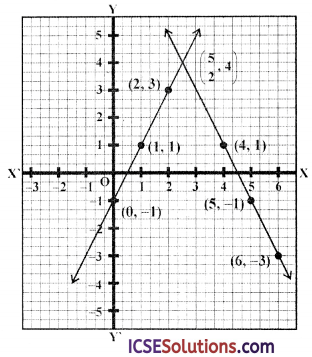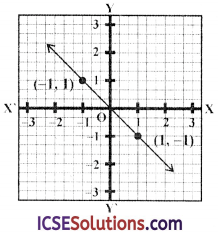Regular engagement with ICSE S Chand Maths Class 9 Solutions Chapter 20 Coordinates and Graphs of Simultaneous Linear Equations Chapter Test can boost students’ confidence in the subject.

## S Chand Class 9 ICSE Maths Solutions Chapter 20 Coordinates and Graphs of Simultaneous Linear Equations Chapter Test

Question 1.
If (1, 2) and (3, 8) are the extremes of a diagonal of a square, then the area of the square is
(a) 10 sq. units
(b) 20 sq. units
(c) 15 sq. units
(d) 8 sq. units
Solution:
(1, 2) and (3, 8) are the end points of a diagonal of a square
∴ Length of diagonal = $$\sqrt{(3-1)^2+(8-2)^2}$$
= $$\sqrt{2^2+6^2}$$ = $$\sqrt{4+36}$$ = $$\sqrt{40}$$
∴ Area of square = $$\frac{(\text { Diagonal })^2}{2}$$
$$\frac{(\sqrt{40})^2}{2}$$ = $$\frac{40}{2}$$ = 20 sq. units (b)Question 2.
If the distance between two points (0, -5) and (x, 0) is 13 units, then x =
(a) 10
(b) ±10
(c) 12
(d) ± 12
Solution:
Distance between (0, -5) and (x, 0) = 13
⇒ $$\sqrt{(x-0)^2+(0+5)^2}$$ = 13
⇒ $$\sqrt{x^2+25}$$ = 13
Squaring both sides,
x2 + 25 = 169 ⇒ x2 = 169 – 25 = 144
⇒ x2 = (± 12)2
∴ x = ± 12 (d)

Question 3.
If A (x, y) is equidistant from P (-3, 2) and Q (2, -3) then
(a) 2x = y
(b) x = -y
(c) x = 2y
(d) x = y
Solution:
A (x, y) is equidistant from P (-3, 2) and Q (2, -3)
Then AP = AQ
⇒ $$\sqrt{(-3-x)^2+(2-y)^2}$$ = $$\sqrt{(2-x)^2+(-3-y)^2}$$
Squaring,
(-3 -x)2 + (2 – y)2 = (2 – x)2 + (-3 -y)2
⇒ 9 + x2 + 6x + 4 + y2 – 4y = 4 + x2– 4x + 9 + y2 + 6y
⇒ 6x – 4y + 13 = 6y – 4x + 13
⇒ 6x + 4x =6y + 4y ⇒ 10x = 10y
∴ x = y

Question 4.
The nearest point from the origin is
(a) (2,-3)
(b) (6,0)
(c) (-2,-1)
(d) (3,5)
Solution:
Distance between origin (0, 0) is
(a) (2, -3) = $$\sqrt{2^2+3^2}$$ = $$\sqrt{4+9}$$ = $$\sqrt{13}$$
(b) (6, 0) = $$\sqrt{6^2+0^2}$$ =$$\sqrt{36}$$ = 6
(c) (-2, -1) = $$\sqrt{(-2)^2+(-1)^2}$$ = $$\sqrt{4+1}$$ = $$\sqrt{5}$$
(d) (3, 5) = $$\sqrt{3^2+5^2}$$ = $$\sqrt{9+25}$$ = $$\sqrt{34}$$
It is clear that point (-2, -1) is nearest to (0, 0) (c)

Question 5.
The coordinates of the vertices of a side of square are (4, -3) and (-1, -5). Its area is
(a) $$2 \sqrt{29}$$ sq. units
(b) $$\frac{\sqrt{89}}{2}$$ sq. units
(c) 89 sq. units
(d) 29 sq. units
Solution:
The coordinates of the each of a side of square are (4, -3) and (-1, -5)
∴ Length of side = $$\sqrt{(-1-4)^2+(-5+3)^2}$$
= $$\sqrt{(-5)^2+(-2)^2}$$
= $$\sqrt{25+4}$$ = $$\sqrt{29}$$
∴ Area of square = (Side)2
= (√29)2 sq. units
= 29 sq. units (d)
Solve by graphing.Question 6.
2x + y = -8
y = $$\frac { 1 }{ 3 }$$x – 1
Solution:
2x + y = -8
y = $$\frac { 1 }{ 3 }$$x – 1
2x + y = -8 ⇒ y = -2x – 8
Giving some different values to x, we get corresponding values of y as given below:

 X -2 -4 -5 y -4 0 2

Now plot the points (-2, -4),(-4, 0),(-5, 2) on the graph and join them to get a line Similarly, y = $$\frac { 1 }{ 3 }$$x – 1

 X 3 6 -3 y 0 1 -2

Plot the points (3, 0),(6, 1) and (-3, -2) on the graph and join them to get another line. We see that these two lines intersect each other at (-3, -2)
∴ x = -3, y = -2Question 7.
Draw the graph of 2x – y – 1 = 0 and 2x + y = 9, on the same axes. Use 2 cm = 1 unit on both axes and plot only 3 points per line. Read the coordinates of their point of intersection.
Solution:
2x – y – 1 = 0 and 2x + y = 9
⇒ x = $$\frac{y+1}{2}$$
Giving some different values of y, we get corresponding values of x as given below :

 X 1 2 0 y 1 3 -1

Plot the points (1, 1),(2, 3) and (0, -1) on the graph and join them to get a line.
Similarly 2x + y = 9
y = 9 – 2x

 X 4 5 6 y 1 -1 -3

Plot the points (4, 1),(5, -1) and (6, -3) on the graph and join them to get another line.
These two lines intersect each other at point $$\left(\frac{5}{2}, 4\right)$$
∴ x = $$\frac { 5 }{ 2 }$$, y = 4Question 8.
Choose the equation whose graph is given here
(a) y = x
(b) x + y = 0
(c) y = 2x
(d) 2 + 3y = 7xSolution:
From the given graph, Two points are given on it whose x and y are equal but in opposite signs
It is through the origin
Equation will be x = -y
⇒ x + y = 0

Question 9.
The distance between the piont (0, 0) and the intersecting point of the graphs of x = 3 and y = 4 is
(a) 4 units
(b) 3 units
(c) 2 units
(d) 5 units
Solution:
The distance between the point (0, 0) and point of intersection of x = 3 and y = 4
i.e., (3, 4) will be = $$\sqrt{(3-0)^2+(4-0)^2}$$
= $$\sqrt{3^2+4^2}$$ = $$\sqrt{9+16}$$
= $$\sqrt{25}$$ = 5 units

Question 10.
The points (-4, 0),(4, 0),(0, 3) are the vertices of a
(a) right triangle
(b) isosceles triangle
(c) equilateral triangle
(d) scalene triangle
Solution:
The points (-4, 0),(4, 0),(0, 3) are given Now,
AB = $$\sqrt{(4+4)^2+(0+0)^2}$$ = $$\sqrt{8^2+0^2}$$ = $$\sqrt{64}$$ = 8
BC = $$\sqrt{(0-4)^2+(3-0)^2}$$ = $$\sqrt{(-4)^2+(3)^2}$$ = $$\sqrt{16+9}$$ = $$\sqrt{25}$$ = 5
AC = $$\sqrt{(0-4)^2+(3-0)^2}$$ = $$\sqrt{(-4)^2+(3)^2}$$ = $$\sqrt{16+9}$$ = $$\sqrt{25}$$ = 5
∴ BC = AC
∴ The given points are the vertices of an isosceles triangle. (b)